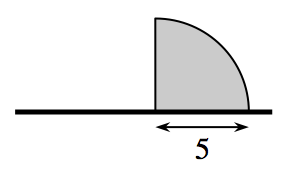Home > APCALC > Chapter 1 > Lesson 1.3.2 > Problem1-120

1-120.

A flag in the shape of a quarter-circle is shown at right.1. Imagine rotating the flag about its pole and describe the resulting three-dimensional figure. Draw a picture of this figure on your paper.

What three-dimensional figure does this shape remind you of?

1. Calculate the volume of the rotated flag.

What is the formula for the volume of a sphere?

How would you determine the volume of half of a sphere?

Use the eTool below to visualize the rotating flag.
Click the link at right for the full version of the eTool: Calc 1-120 HW eTool